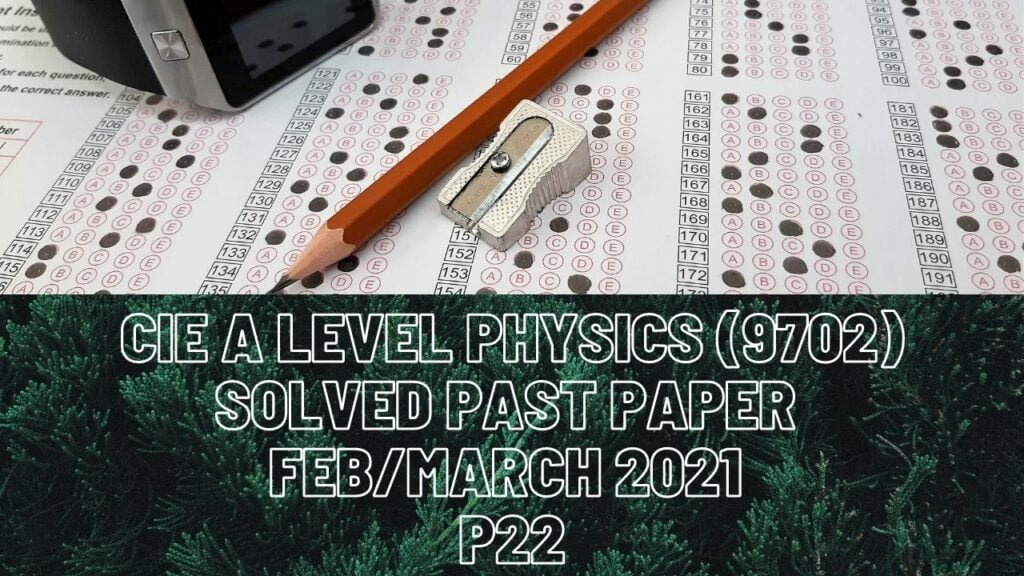A Level Physics### CIE A Level Physics Solved Past Paper Feb/March 2021 P22

1 cii) Force is directly proportional to acceleration which is constant for the first and last four seconds so constant resultant force for both these intervals except that resultant force for the last four seconds will be greater and on the negative axis due to greater negative acceleration. For t=4 to t=8s, the acceleration is zero so zero resultant force.

2 bii) g.p.e. loss, which is 60J, is the g.p.e. that the ball has when it is at the height of the balcony. This energy converts to some kinetic energy with which it lands on the ground level (23J) and some energy that is lost in the form of heat to the surroundings.

c) Whenever a Q is dealing with terminal velocity, the object always starts to fall with a=9.81m/s^2 which decreases over time because air resistance increases with the ball’s speed. As a result, the resultant force on the ball starts to decrease which means the acceleration will decrease to zero at the terminal velocity state.

3 ci) For an object that is in an equilibrium state, all the forces that are acting on it must be balanced. In this case, the weight of the cylinder must be equal to the upthrust on the cylinder due to the liquid + force exerted by the spring at that particular point (extension of 4cm).

ii) Upthrust on cylinder is always due to difference in pressure at the top and bottom faces of the cylinder.

di) Increase in the density of liquid means an increase in the pressure difference between the top and bottom faces of the cylinder that in turn increases the upthrust due to liquid on the base of the cylinder. When the upthrust increases, to keep the equation valid (weight=upthrust+force due to spring at extension 4cm), the force due to the spring at extension 4cm must decrease. This force due to spring is directly proportional to the extension of the spring (F=kx) so extension decreases.

4 bi) To form a stationary wave, we must have incoming and reflected waves both overlapping each other so a reflector is used for that.

iii) The wave pattern of stationary waves and the length of them are fixed for both kinds of air columns that you study in AS Level Physics. Here, the column will have an open end and a closed-end so an anti-node on the open end and a node on the close end because the reflector is the closed-end and the receiver is the open end. The Q has asked you to find the distance from X when the next possible minimum is detected.

6 bii) Since the lamp and resistor are connected in a parallel loop, p.d. across the lamp is equal to p.d. across the resistor. The e.m.f. is 12V but the p.d. is not 12V; Instead, it’s only 4.4V which means the difference between them was lost due to the battery’s internal resistance.

iv) Average drift speed of free electrons is inversely proportional to area and inversely proportional to squared radius.